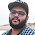# Variables and Data types in dart

Variables are containers for data. You can say variables are a box in which any data is stored and now the data is known as the box (The name of the variable).
Variable is the "name of memory block" which means a variable is a named space booked in memory.

## How to define a variable

First, you should understand what it means to "define a variable". Let me clear, The process of storing data into the variable is called "Defining a variable".

Defining a variable called "name"
To define a variable we should have three things
1. Data type
2. Variable Name (Identifier)
3. Data (Value or reference)

### Data type:

In the Example above var is a Data Type. But Wait..... What is a data type, Data type is nothing but "The type of data which we are going to store in the variable". There are the following types of data-type in the Dart programming language.

• Numbers
• Strings
• Booleans
• Lists
• Maps

### Numbers:

Numbers in dart is used to represent integer values, There are two types of numbers:

(1) Integer:

Integers in dart are used for non-fractional data. I.E. numeric value without a decimal point. For example, "22" is an Integer because it has not any decimal point, similarly "-22" is also an integer. We define Integer data types with int keyword.
` int a = 12; `

(II) Double:

Doubles in dart are used for fractional data. I.E. values decimal points. For example, "12.4" is double type, similarly "-26.3" is also a double type. We define double Data type with double keyword.
` double a = 14.4; `
` double b =  -12.2; `

### String:

Strings in dart are used to represent a character or sequence of characters. For example, if you are data i.e. name, address, etc then that is String type. All the string type data must be enclosed with a single quote, double quote, or even triple quota in some places. We define string Data type with String keyword.

` string a = "edslide"; `

### Booleans:

Booleans are used for representing true, false values. Booleans are defined with the bool keyword.
` bool a = true; `

### Lists:

The list data type is used for representing a list of data For example, All the students of a class, All the items in a menu. etc. Lists are like "Array" in many programming languages. We will discuss further on lists in thins tutorial.

### Maps:

Maps are nothing but some data with key-value pairs. For example, a rate card in a shop with a key-value pair of items and price. We will discuss further on lists in thins tutorial.

So these are the data types in the dart. But earlier in an example I used the "var" keyword instead of these Data types. What is the "var" keyword, let's discuss this.

### Identifiers

Identifiers in dart are the variable name in which we are assigning the value. For example, String a = "Hello"; here "a" is Identifier. There are some rules applied to the naming of identifiers :

### variable initialization

While coding you will often hear a word variable initialization.  So what is the variable initialization lets talk about this, Variable initialization is the process of how a variable is born, which means the first step of creating a variable. For example,  String a  = "edslide";  means variable a ins initialized with value "edslide". In variable, it is not compulsory to assign any value to the variable like this      String a;  when you do not assign any value to the variable by default null will be assigned to the variable.

### The var keyword in dart :

var keyword in dart is used for automatic declaration of a data type. By using var you don't have to declare datatype, just write the var keyword instead. For Example
` var a = "edslide"; `
` var b = 2; `
` var c = 2.2; `

### The dynamic keyword in dart:

dynamic keyword in dart is the same as var keyword with a minor change. In var keyword, if you initialized(first-time data inserted) any specific data then you cannot use another data type. For example, if a variable named a is initialized with "edslide"  var a = "edslide";     then you cannot assign any integer or boolean or any other data type, for example,  var a = 2;  will give you an error.
But here comes dynamic keyword to rescue by using the dynamic keyword you will not get any error.
` dynamic a = "edslide" `

` a = 2; `

` a = 2.2; `

1.